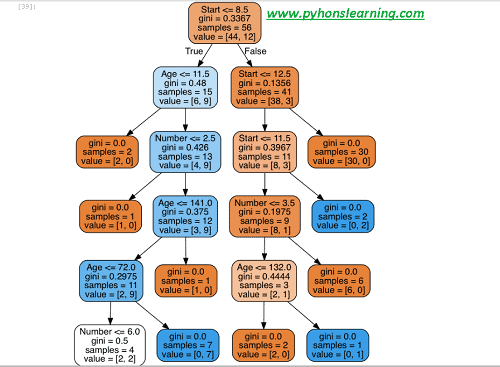# Decision Trees and Random Forests classifier in Python

Welcome everyone, Today we will see Decision Trees and Random Forests classifier-and Types in Python so let’s start:

In this project following steps are used to performed operation:
• Import algorithm Decision Trees and Random Forests classifier package.
• Get the data
• Split data into x/y_training and x/y_test data.
• Train or fit the data into the different model methods.
• Prediction and Evaluation the data
• Decision Trees visualization
• Random Forests
• finally generate the Tree (learn different python terminologies)

## Import algorithm Decision Trees and Random Forests classifier package

`Import Librariesimport pandas as pdimport numpy as npimport matplotlib.pyplot as pltimport seaborn as sns%matplotlib inline`

Get the Data

`df = pd.read_csv('Decision Trees.csv')`

`Decision  Age Number Start0 present 34 3 91 absent 58 4 152 absent 28 5 83 present 72 3 44 absent 81 4 15`

### Split data into x/y_training and x/y_test data.

Let’s start to split up the data into a training and test set.

`from sklearn.model_selection import train_test_splitx = df.drop('Decision Trees',axis=1)y = df['Decision Trees']x_train, x_test, y_train, y_test = train_test_split(x, y, test_size=0.20)`

#### check out Decision Trees

We will start just by training a single decision trees in this section.

`from sklearn.tree import DecisionTreeClassifierdtree = DecisionTreeClassifier()dtree.fit(x_train,y_train)`
`DecisionTreeClassifier(class_weight=None, criterion='gini', max_depth=None,            max_features=None, max_leaf_nodes=None, min_samples_leaf=1,            min_samples_split=2, min_weight_fraction_leaf=0.0,            presort=False, random_state=None, splitter='best')`

How to Prediction and Evaluation the data
Let’s start to evaluate our decision tree.

`predictions = dtree.predict(x_test)from sklearn.metrics import classification_report,confusion_matrixprint(classification_report(y_test,predictions))`

`         precisionx    recallx  f1-scorex   supportx    present       0.80      0.80      0.80        15    absent       0.45      0.45      0.45         10avg / total       0.75      0.75      0.75        25`

`print(confusion_matrix(y_test,predictions))[[18  4] [ 2  3]`

Tree Visualization

`from IPython.display import Image  from sklearn.externals.six import StringIO  from sklearn.tree import export_graphvizimport pydot `

`features = list(df.columns[1:])features['Ages', 'Numbers', 'Starts']dot_data = StringIO()  export_graphviz(dtree, out_file=dot_data,feature_names=features,filled=True,rounded=True)graph = pydot.graph_from_dot_data(dot_data.getvalue())  Image(graph.create_png())  `Decision Trees and Random Forests classifier-Types in Python

Random Forests
Now This time to compare the decision tree model to a random forest.

`from sklearn.ensemble import RandomForestClassifierrfc = RandomForestClassifier(n_estimators=100)rfc.fit(x_train, y_train)`

`RandomForestClassifier(bootstrap=True, class_weight=None, criterion='gini',            max_depth=None, max_features='auto', max_leaf_nodes=None,            min_samples_leaf=1, min_samples_split=2,            min_weight_fraction_leaf=0.0, n_estimators=100, n_jobs=1,            oob_score=False, random_state=None, verbose=0,            warm_start=False)rfc_pred = rfc.predict(X_test)`

`print(confusion_matrix(y_test,rfc_pred))[[14  3] [ 2  4]]print(classification_report(y_test,rfc_pred))`

`             precisionx    recallx  f1-scorex   supportx    present     0.83      0.80      0.78        10    absent      0.55      0.50      0.54         15avg / total       0.75      0.70      0.77        25`

How many types of decision trees in machine learning?
It’s based on the type of target variable in python.
1. Categorical variable Decision Tree: If in Decision Tree which has a available categorical target variable then it called a Categorical variable decision tree in python.
Summary:
In this section we saw the Decision Trees and Random Forests classifier-Types in Python. About this section you have any query then please comment
Tags:
Decision Trees and Random Forests classifier-Types in Python, python, machine learning
If you want to machine learning interview question then click on it.
BEST OF LUCK!!!

### sachin PagarI am Mr. Sachin pagar Embedded system engineer, the founder of Pythonslearning, a Passionate Educational Blogger and Author, who love to share the informative content on educational resources.

### sachin Pagar

I am Mr. Sachin pagar Embedded system engineer, the founder of Pythonslearning, a Passionate Educational Blogger and Author, who love to share the informative content on educational resources.